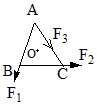Questions

# ‘O’ is the centre of an equilateral triangle ABC. F1, F2 and F3 are three forces acting along the sides AB, BC and AC as shown in figure. What should be the magnitude of F3, so that the total torque about ‘O’ is zero ?Easy
A

$\frac{{F}_{1}+{F}_{2}}{2}$

B

$2\left({F}_{1}+{F}_{2}\right)$

C

$\left({F}_{1}+{F}_{2}\right)$

D

$\left({F}_{1}-{F}_{2}\right)$